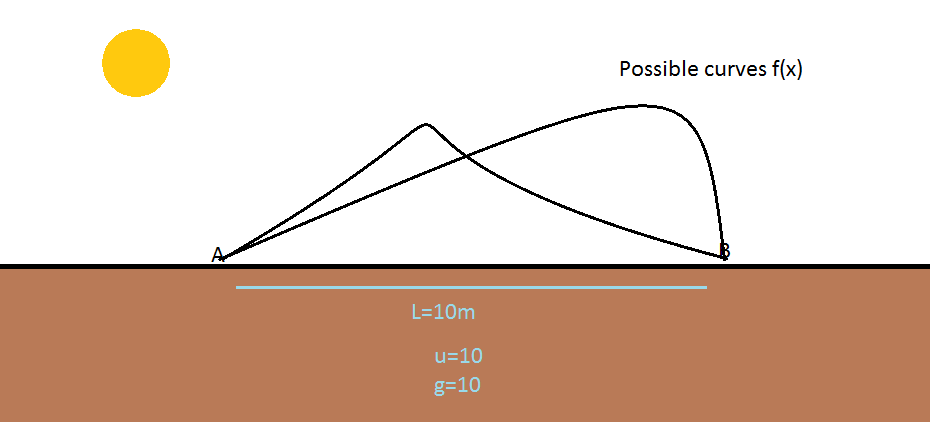# Is it hard?Consider all the possible continuous smooth 2-D curves joining two points on the ground ( 'A' and 'B' ) seperatedd by a distance L and lying entirely in the vertical plane,

Let the curve be described as y=f(x) satisfying f'(0)=1
and also as has been mentioned f(0)=f(L)

A particle is to be launched along the curve with an initial speed of u from A, so as to reach at B,

What is the equation of the curve f(x) so that the curve exerts absolutely no Normal reaction upon the particle at each and every point on the curve,

if it can be expressed as

$y=Ax+B{ x }^{ 2 }+C{ x }^{ 3 }+D{ x }^{ 4 }+E{ x }^{ 5 }.......\quad$

Find the sum of the coefficients (algebraic sum , not sum of magnitudes)

Details and Assumptions

1) L= 10

2) g= 10

3) u= 10

3) Neglect buoyancy , viscous drag , size of particle and variation of 'g' with height

(ALL IN SI UNITS)

×

Problem Loading...

Note Loading...

Set Loading...